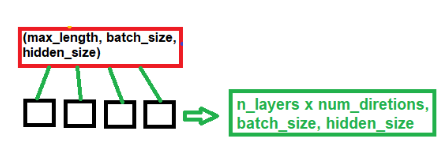# Retrieving hidden and cell states from LSTM in a Language model

What is the order of the hidden and cell states in the tuple that is returned by LSTM? Particularly, in the word LM model, in `generate.py` , we have:

``````with open(args.outf, 'w') as outf:
with torch.no_grad():  # no tracking history
for i in range(args.words):
output, hidden = model(input, hidden)
....
......
``````

In the above code, `hidden` is a tuple with two tensors, each of same shape. Since this is an LSTM, it returns both `h` and `c` states. But, how is `h` and `c` ordered in the tuple?

My understanding is this:

``````h, c = hidden
``````

Is this correct? Or is it the other way around?

Thanks!

It’s correct if it’s not an LSTM Cell. If you’r using `nn.LSTM`, then this would return `output, (h_n, c_n)`, however if your using `nn.LSTMCell`, then this would return `h_1, c_1`. The order is the hidden state and then the cell state. So in your case, `hidden` would be equal to `(h_n, c_n)`.

1 Like

Thank you!

Yes, I’m using `nn.LSTM` with 2 hidden layers (unidirectional). So, I also want to ask about the shape of `h_n` specifically in this case. For example, I get the shape of `h_n` as `torch.Size([2, 1, 1500])`

If I understand it correctly, I can get the hidden states of the hidden layers by indexing into them in the order:

``````first_hidden_layer_hidden_state = h_n    # torch.Size([1, 1500])
second_hidden_layer_hidden_state = h_n[-1]    # torch.Size([1, 1500])
``````

Is this a correct way of indexing and getting the hidden states?

P.S.: I’m just interested only in these hidden states because I want to pass them to a downstream network for further processing.

Hi. The outputs for the LSTM is shown in the attached figure.The output shape for `h_n` would be `(num_layers * num_directions, batch, hidden_size)`. This is basically the output for the last timestep. Your output is `(2,1,1500)` so you are using 2 layers*1 (unidirectional) , 1 sample and a hidden size of 1500).
Now the LSTM would return for you `output`, `(h_n, c_n)`. In your case, `(h_n, c_n)` is named `hidden.` So by indexing hidden you can extract the `h_n` and `c_n` (i.e `hidden = h_n` and `hidden = c_n`)

1 Like

Thank you for clear explanation. It was very helpful! I just want to confirm one point again:

Since I’m using 2 layer LSTM network, I want to extract hidden state of the last hidden layer. Is the following way of indexing correct way to do:

first_hidden_layer_hidden_state = h_n # torch.Size([1, 1500])
second_hidden_layer_hidden_state = h_n[-1] # torch.Size([1, 1500])

If the above is correct, I’m interested in `second_hidden_layer_hidden_state`.

Also, is it analogous for the cell state as well?

first_hidden_layer_cell_state = c_n # torch.Size([1, 1500])
second_hidden_layer_cell_state = c_n[-1] # torch.Size([1, 1500])

Thank you!

To retrieve the hidden or cell states of the last (or any of the) hidden layers, the diagram in whats-the-difference-between-hidden-and-output-in-pytorch-lstm is very helpful and makes it easy to understand.

In short, the number ranges from [0, 1, 2, 3, …, n] for [hidden_layer_1, hidden_layer_2, hidden_layer_3, …, hidden_layer_n] for `n` hidden layers.

So, with two hidden layers, the `hidden` and `cell states` of the second (or last) hidden layer can be extracted using:

analogously for the `cell state`:

1 Like